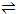Explain the mechanism of Enzyme Reactions.

A mechanism for enzyme-catalyzed reactions that leads to the typical rate equation for these reactions can be described.

A variety of rate equations are required to portray the rates of enzymes catalyzed reagents and physical conditions that are encountered. The rate equation of however, is a guide to many of these variations, and the mechanisms of this section, often called the Michaelis-Menten mechanism, is likewise a base for other variations.

The mechanism that accounts for the rate equation is similar to those dealt in with.

With S representing substrate, E the enzyme, and E. S and enzyme substrate complex, the mechanism is presumed to be adequately represented by

E + SE. S

E. SE + products

The steady state assumption, which, however, is not always clearly applicable in these reactions, leads to

k1[E][S] = k-1[E. S] + k2[E. S]

And [E. S] = k1/k-1 + k2 [E][S]

To bring these expressions to a form that can be compared with the empirical rate equation, we must recognize that only [Etot] = [E] + [E. S], and not [E], is generally known. Often, in fact, only a quantity proportional to [Etot], and not even values of [Etot], is available.

Replacement of [E] in equation by [E] = [Etot] - [E. S] leads to

[E. S] = k1[Etot][S]/(k-1 + k2) + k1[S]

Now this expression for the intermediate E. S can be inserted into the expression for the rate of the net reaction. This rate can be based on the formation of products in the second mechanism step. We have

-d[S]/dt = R = k2[E. S] = k1k2[Etot][S]/k-1 + k2 + k1[S]

= k2[Etot][S]/k-1 + k2)/k1 + [S]

It is customary for the term (k-1 + k2)/k1 to be obtained by the new symbol KM, that is,

KM = k-1 + k2/k1 to give the rate equation result of this mechanism as

R = k2[Etot][S]/KM + [S]

We have come at this stage to the form of the empirical rate equation obtained, we are now in a position to intercept the values of the parameters KM and k2[Etotin terms of their roles in the roles in the steps of the mechanism.

Reference to equation shows that, as the reaction is proceeding

[E][S]/[E. S] = KM

Thus KM is related to species concentrations, as is the dissociation equilibrium constant for the species [E. S]. the value of KM, however, is given by (k...1 + k2)/k1, and this equal to the value of the dissociation constant for [E. S] only to the extent that k2 is small and can be neglected compared with k...1. Thus when the breakup of the E. S complex to form original E and S species dominates the process whereby the complex forms products, the value of KM approaches the dissociation constant for the E. S complex.

What, now, is the significance of the term k2[Etot]? One first notes that the rate of the overall reaction is

R = k2[E. S]

It follows that k2[Etot] is the rate that the reaction would have if all the enzyme were in the form of the enzyme-substrate complex. Thus k2[Etot] is the maximum rate for a given value of [Etot]. The turnover rate of an enzyme in a particular enzyme-catalyzed reaction is the rate per mole of enzyme, i.e. the turnover rate is equal to the value of k2, and this can be calculated fromk2[Etot] if the total enzyme concentration is known.

#### Related Questions in Chemistry

• ##### Q :Theory of one dimensional motion For

For motion in one dimension, the distribution of the molecules over quantum states, speeds, and energies can be deduced.Here we show that the energy of a macroscopic gas sample can be described on the basis of our knowledge of the quantum states allowed to

• ##### Q :Problem on making solution Select the

Select the right answer of the question. The weight of H2C2O42H2O required to prepare 500ml of 0.2N solution is : (a) 126g (b) 12.6g (c) 63g (d) 6.3g

• ##### Q :Molar mass lculwhat is the equation for

lculwhat is the equation for caating molar mass of non volatile solute

• ##### Q :Molecular weight of substance The

The boiling point of a solution of 0.11 gm of a substance in 15 gm of ether was found to be 0.1oC higher than that of the pure ether. The molecular weight of the substance will be (Kb = 2.16)       (a) 148 &nbs

• ##### Q :Problem on equilibrium constant Ethanol

Ethanol is manufactured from carbon monoxide and hydrogen at 600 K and 20 bars according to the reaction2 C0(g) + 4 H2(g) ↔ C2H5OH(g) + H2O (g)The feed stream contains 60 mol% H2, 20 m

• ##### Q :Describe First Order Rate Equation The

The integrated forms of the first order rate equations are conveniently used to compare concentration time results with this rate equation. Rate equations show the dependence of the rate of the reaction on concentration can be integrated to give expressions fo

• ##### Q :Linde liquefaction process Liquefied

Liquefied natural gas (LNG) is produced using a Linde liquefaction process from pure methane gas at 3 bar and 280 K (conditions at point 1 in figure below). A three-stage compressor with interceding is used to compress the methane to 100 bar (point 2). The first stage

• ##### Q :Question on seminormal solution Provide

Provide solution of this question. The weight of sodium carbonate required to prepare 500 ml of a seminormal solution is: (a) 13.25 g (b) 26.5 g (c) 53 g (d) 6.125 g

• ##### Q :How haloalkanes are prepared from

This is the common method for preparing haloalkanes in laboratory. Alcohols can be converted to haloalkanes by substitution of - OH group with a halogen atom. Different reagents can be used to get haloa

• ##### Q :The three facts on the evaporation

Describe briefly the three facts on the evaporation?